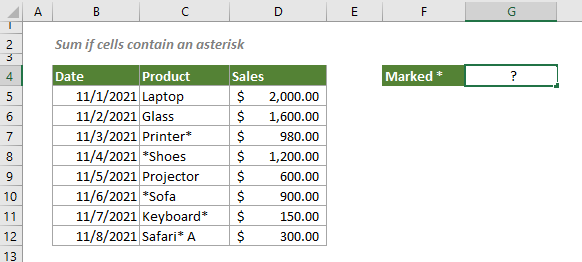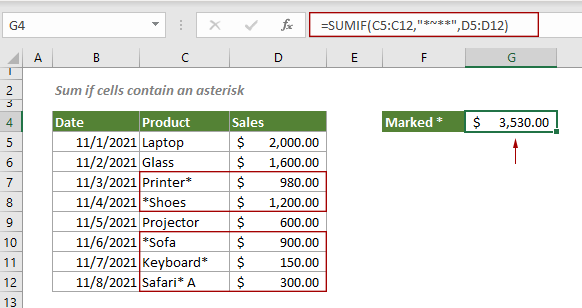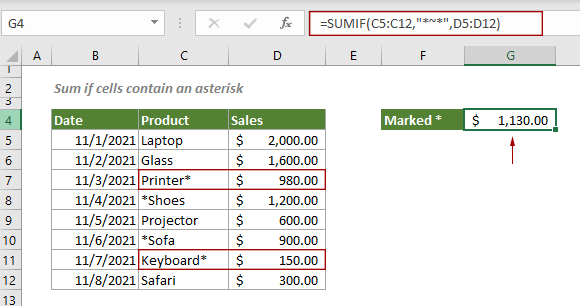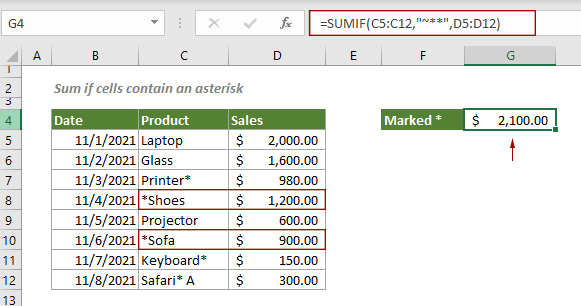## Sum if cells contain an asterisk in Excel

As we all know, the asterisk is a wildcard in Excel. To sum cells containing an asterisk that is regarded as a literal character not a wildcard, you can apply a formula based on the SUMIF function and the tilde (~).

#### How to sum if cells contain an asterisk in Excel?

As shown in the screenshot below, to sum sales in the range D5:D12 when the corresponding cell in range C5:C12 contains an asterisk, you can follow the steps below.Generic Formulas

=SUMIF(range,"*~**",[sum_range])

Arguments

Range: A range of cells which you want to evaluate if it contains an asterisk;
Sum_range: A range of cells that you want to sum.

How to use this formula?

Select a cell to output the result, copy or enter the below formula and press the Enter key.

=SUMIF(C5:C12,"*~**",D5:D12)Notes: The above formula sums cells no matter where the asterisk is in a cell.

To sum cells whose asterisk is at the end of the cell, you can apply a formula like this:

=SUMIF(C5:C12,"*~*",D5:D12)To sum cells whose asterisk is at the beginning of the cell, apply the formula below:

=SUMIF(C5:C12,"~**",D5:D12)Explanation of the formula

=SUMIF(C5:C12,"*~**",D5:D12)

ROW(E5:E14)-ROW(E5)+1:
> "*~**": To prevent Excel from treating asterisks as wildcards, you can add a tilde (~) before an asterisk to mark this asterisk as a literal character. Here the first and the last asterisk is a wildcard which means “one or more characters”; the asterisk after the tilde represents a literal asterisk.
The whole “*~**” means to match all cells containing an asterisk, no matter where the asterisk is in a cell.

#### Related functions

Excel SUMIF function
The Excel SUMIF function can help to sum cells based on one criterion

#### Related formulas

Sum if cells contain both x and y
This step-by-step tutorial demonstrates how to sum a range of cells if the corresponding cells containing both x and y by using the SUMIFS function.

Sum if cells contain or equal to either x or y
To sum the cells if contain or equal to either one value or another, this tutorial provides two formulas in detail to help solve the problems.

Sum if date is between two dates
This tutorial demonstrates how to sum values within a certain date range in Excel with a formula based on the SUMIFS function.

Sum if cells are equal or not equal to a certain value
This step-by-step tutorial explains two formulas to sum a range of cells that equal or not euqal to a certain value based on the SUMIF function.

### The Best Office Productivity Tools

#### Kutools for Excel - Helps You To Stand Out From Crowd

 Popular Features: Find, Highlight or Identify Duplicates  |  Delete Blank Rows  |  Combine Columns or Cells without Losing Data  |  Round without Formula ... Super VLookup: Multiple Criteria  |  Multiple Value  |  Across Multi-Sheets  |  Fuzzy Lookup... Adv. Drop-down List: Easy Drop Down List  |  Dependent Drop Down List  |  Multi-select Drop Down List... Column Manager: Add a Specific Number of Columns  |  Move Columns  |  Toggle Visibility Status of Hidden Columns  |  Compare Columns to Select Same & Different Cells ... Featured Features: Grid Focus  |  Design View  |  Big Formula Bar  |  Workbook & Sheet Manager | Resource Library (Auto Text)  |  Date Picker  |  Combine Worksheets  |  Encrypt/Decrypt Cells  |  Send Emails by List  |  Super Filter  |  Special Filter (filter bold/italic/strikethrough...) ... Top 15 Toolsets:  12 Text Tools (Add Text, Remove Characters ...)  |  50+ Chart Types (Gantt Chart ...)  |  40+ Practical Formulas (Calculate age based on birthday ...)  |  19 Insertion Tools (Insert QR Code, Insert Picture from Path ...)  |  12 Conversion Tools (Numbers to Words, Currency Conversion ...)  |  7 Merge & Split Tools (Advanced Combine Rows, Split Excel Cells ...)  |  ... and more

Kutools for Excel Boasts Over 300 Features, Ensuring That What You Need is Just A Click Away...#### Office Tab - Enable Tabbed Reading and Editing in Microsoft Office (include Excel)

• One second to switch between dozens of open documents!
• Reduce hundreds of mouse clicks for you every day, say goodbye to mouse hand.
• Increases your productivity by 50% when viewing and editing multiple documents.
• Brings Efficient Tabs to Office (include Excel), Just Like Chrome, Edge and Firefox.No ratings yet. Be the first to rate!
This comment was minimized by the moderator on the site
Hi Crystal;
I tried it in Microsoft365 and Excel2010.
Thanks
This comment was minimized by the moderator on the site
That is cool. Thank you.
However, I was looking for something else.
I have certain numbers that I have to add in a column that have asterisks.
For example: The column has numbers 8.00, 7.00*, 40.00, 10.00*; which equals, 65.00. The numbers with the asterisks do not add (of course) so the sum = 48.00.
So, is there a way to have the asterisks and have it add them too?
Thanks a bunch!
Ressa
This comment was minimized by the moderator on the site
Hi Ressa,
The following array formula can help. Suppose your numbers are in range A2:A6, after entering the formula in a cell, press the Ctrl + Shift + Enter keys on your keyboad to get the result.
=SUM(IF(ISNUMBER(SEARCH("*",A2:A6)),SUBSTITUTE(A2:A6,"*","")+0))
This comment was minimized by the moderator on the site
Thank you Crystal,
It always returns zero when I use it.
Does it replace the "*" with nothing?
They want the "*" still there.
I am sure there is no way to have a formula.
Thanks,
Ressa
This comment was minimized by the moderator on the site
Hi Ressa,
This formula works well in my case. May I ask which Excel version are you using?
There are no comments posted here yet